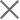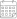Subscribe

### Indexes

Quarter 1-2, 2020 | Energy Market Review08 February 2021

In the first and second quarters of 2020, Georgian power plants generated 2,893 mln. and 2,797 mln. kWh of electricity, respectively (Figure 1). This represents a 1.5% and 9.7% decrease in total generation compared to the corresponding periods of the previous year (in 2019, the total generation in Q1 was 2,936 mln. kWh and 3,097 mln. kWh in Q2). On an annual basis, the reduction in generation in the first quarter of 2020 was derived from a 19.3% and 2.7% decline in hydro and wind power generation, respectively, offsetting the 28.3% annual increase in thermal power generation. The decrease in production in the second quarter is, instead, related to the 46.2% and 1.2% decrease in thermal and hydropower generation, more than offsetting the 10% increase in wind power generation.

In the first two quarters of 2020, electricity consumption on the local market amounted to 3,491 mln. (Q1) and 2,883 mln. kWh (Q2) (+3.9% and -8% compared to the first and second quarters of 2019, respectively) (Figure 1). Moreover, power consumption exceeded generation by 598 mln. and 86 mln. kWh, 20.7% and 3.1% of total generation, respectively (in 2019 the difference between total generation and consumption resulted in a shortage in the first quarter of 423 mln. kWh, around 14.4% of the total generation, and 38 mln. kWh, around 1.2% of the total generation, in the second quarter).